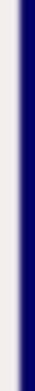homework help forum today's math problem links utilities previous math problem feedback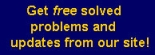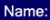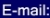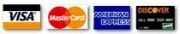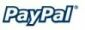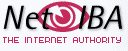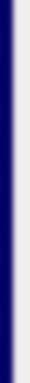# MATH HOMEWORK HELP

11. Find the equation of the tangent to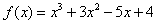at x=1.

Solution: We first differentiate the function to get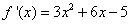and evaluating at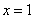, we find that the slope is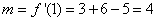Finally, the equation of the tangent line is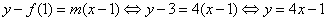MGT - Your source of Math and Statistics Homework Help